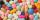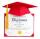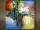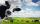Runner 2

Marian run 12 meters in 8 seconds. How far would Marian run for 70 seconds, if still run at the same pace?

Result

x =  105 m

Solution:Leave us a comment of this math problem and its solution (i.e. if it is still somewhat unclear...):Be the first to comment!Next similar math problems:

1. RunnersFor three runners (on the first to third place) is prepared 30 chocolate that they be distributed in the ratio of 3 : 2 : 1 How much chocolate will get everyone?
2. Masons1 mason casts 30.8 meters square in 8 hours. How long casts 4 masons 178 meters square?
3. PillsIf it takes 20 minutes to run a batch of 100 pills how many minutes would it take to run a batch of 50 pills
4. Train delayThe train should be in the station for 10 minutes. It still has 32 km left. What is the expected delay if the train travels in 2 minutes 3 km except for the last section where it is 2 km in 5 minutes.
5. Cars 6At 9:00 am two cars started from the same town and traveled at a rate of 35 miles per hour and the other car traveled at a rate of 40 miles per hour. After how many hours will the cars be 30 miles apart?
6. DiplomasSuzanne helped write out diplomas. Issuance its first took 3 minutes, each next 2.3 minutes. How much time it will take to write out n=16 diplomas (including the first), write out this pace?
7. DonutsFind how many donuts each student will receive if you share 126 donuts in a ratio of 1:5:8
8. Image scaleThe actual image dimensions are 60 cm x 80cm and has a reduced size 3 cm x 4 cm. At what scale the image was reduced?
9. Line segmentThe 4 cm long line segment is enlarged in the ratio of 5/2. How many centimeters will measure the new line segment?
10. Land areaA land area of Asia and Africa are in a 3: 2 ratio, the European and African are is 1:3. What are the proportions of Asia, Africa, and Europe?
11. Hour salaryYou work for 4 hours on a Saturday and 8 hours on Sunday. You also receive a \$50 bonus. You earn \$164. How much did you earn per hour?
12. PeppersIn the box are yellow (a), green (b) and red (c) peppers. Their amount is in a ratio 2:4:1 . Most are yellow peppers and green the least. Calculate the number of peppers each type if the total number of peppers is 70.
13. AgesJohn, Teresa, Daniel and Paul have summary 56 years. Their ages are in a ratio of 1:2:5:6. Determine how many years have each of them.
14. Two numbersFind two numbers whose difference and ratio is 2.
15. Cows4 cows spent 16 bags of hay in 5 days. How many bags of hay sacks is needed for 5 cows for seven days?
16. 1.5 divided1.5 divided by 1 = w divided by 4
17. Round-tripA woman works at a law firm in city A which is about 50 miles from city B. She must go to the law library in city B to get a document. Find how long it takes her to drive​ round-trip if she averages 40 mph.